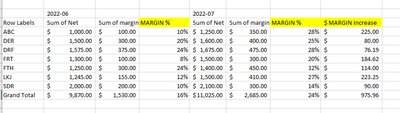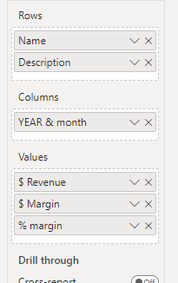cancel
Showing results for
Did you mean:Frequent Visitor

## Calculating % increase as dollar value - DAX

Hello,

I'm relatively new to powerBI and I'm trying to calculate the actual \$ amount of margin increase compared to the margin % last month, and I'm struggling with the DAX (Therefore as we have increase the margin %, how much increase in margin have we generated).

Wondering if anyone can help, I am using the following measures in a Matrix table

Total Revenue - Measure : TOTAL Revenue = sum(Table1[Net])

Total Margin - Measure : Total Margin = Table1[Measure : TOTAL Revenue]-Table1[Measure : Total Oncosts]

Total Margin % - Measure : Margin % = divide(Table1[Measure : Total Margin],Table1[Measure : TOTAL Revenue],0)

In excel I have calculated the margin \$ amount to be

Current Revenue * (current month Margin % - Last months Margin %)

I've been trying to use previous month etc.... but its not working, can anyone help write the correct DAX? I've attached an example in excel of what I am trying to write. can anyone assist?Current set up on my matrix is -That has slicers that can change the months

1 ACCEPTED SOLUTIONSuper User

``````Prior Month % =
CALCULATE (
[Measure : Margin %],
Table1[YearMonth Sequential Number]
= MAX ( Table1[YearMonth Sequential Number] ) - 1,
ALL ( Table1[Year & Month] )
)``````
9 REPLIES 9Super User

Hi @Natty004
First create the year month sequential number number (Calculated Column)

``````YearMonth Sequential Number =
RANKX (
'Date',
YEAR ( 'Date'[Date] ) * 100
+ MONTH ( 'Date'[Date] ),
,
ASC,
DENSE
)``````

Then the required measure would be

``````\$ MArgine Increase =
VAR CurrentMonth =
MAX ( 'Date'[YearMonth Sequential Number] )
VAR CurrentMonthRevenue = [TOTAL Revenue]
VAR CurrentMonthMarginPercent = [Margin %]
VAR PreviousMonthMarginPercent =
CALCULATE ( [Margin %], 'Date'[YearMonth Sequential Number] = CurrentMonth - 1 )
RETURN
CurrentMonthRevenue * ( CurrentMonthMarginPercent - PreviousMonthMarginPercent )``````Frequent Visitor

Hello tamerji,

Thanks for your response. I have added the above calculations and measures however the

\$ margin increase amount is the same value as margin amount.

See below:

``````YearMonth Sequential Number =
RANKX (
'Table1',
YEAR ('Table1'[Dt Invoice]) * 100
+ MONTH ( 'Table1'[Dt Invoice] ),
,
ASC,
DENSE
)``````

Measure

``````\$ MArgine Increase =
VAR CurrentMonth =
MAX ( Table1[YearMonth Sequential Number] )
VAR CurrentMonthRevenue = 'Table1'[Measure : TOTAL Revenue]
VAR CurrentMonthMarginPercent = [Measure : Margin %]
VAR PreviousMonthMarginPercent =
CALCULATE([Measure : Margin %],Table1[YearMonth Sequential Number]=CurrentMonth-1)
RETURN
CurrentMonthRevenue * ( CurrentMonthMarginPercent - PreviousMonthMarginPercent )``````

Margin \$ measure

``Measure : Total Margin = Table1[Measure : TOTAL Revenue]-Table1[Measure : Total Oncosts]``

I then tried to calculate current margin % and Prior month Matgin % and add these to the matrix to see if they are calculating correctly.

The current margin % measure below matches the same calculation [Measure : Margin %]

``````Current Month % = CALCULATE(
divide(Table1[Measure : Total Margin],
Table1[Measure : TOTAL Revenue],0),
Table1[YearMonth Sequential Number])``````

Prior month % - does not pull through the previous month - it just duplicates the current month %

``````Prior Month % =
VAR CurrentMonthMargin = CALCULATE([Measure : Margin %], Table1[YearMonth Sequential Number])
VAR PriorMonthMargin = CALCULATE([Measure : Margin %], Table1[YearMonth Sequential Number] -1)
RETURN
PriorMonthMargin``````

Apologies in advance, are you able to identfy where I am going wrong?Super User

``````Prior Month % =
CALCULATE (
[Measure : Margin %],
Table1[YearMonth Sequential Number]
= MAX ( Table1[YearMonth Sequential Number] ) - 1,
ALL ( Table1[Year & Month] )
)``````Frequent Visitor

Thank you @tamerj1 - your assitance is greatly appreciatedFrequent Visitor

Thanks @tamerj1  for your response. Unfortunately didnt resolve the issue.

The issue I'm trying to resolve is to calculate the monetary amount to the margin increase applied.

Within Excel the formula is = CURRENT MONTH REVENUE * (CURRENT MONTH MARGIN % - Previous Month margin %).

I’m presenting the data in a MATRIX table – so that the user can select the correct months.

List of measures:

TOTAL \$ Revenue = sum('MASTER DATA'[Net])

Total \$ Oncosts = sumx('MASTER DATA',('MASTER DATA'[cost 1]+'MASTER DATA'[cost 2]+'MASTER DATA'[Other 1]+'MASTER DATA'[Other 2]+'MASTER DATA'[cost 3]+'MASTER DATA'[cost 4])*'MASTER DATA'[Bill UTY])

Total \$ Margin = [TOTAL \$ Revenue]-[Total \$ Oncosts]

Margin % = divide('PIP Measures'[Total \$ Margin],'PIP Measures'[TOTAL \$ Revenue],0)

I've added - Year Month sequential number

The measure below is returning the same amount as Total \$ Margin - I cant seem to work out why its going wrong?🤔

Margin \$ Increase - V2 =

VAR CurrentMonth =

MAX ( 'MASTER DATA'[YearMonth Sequential Number] )

VAR CurrentMonthRevenue = 'PIP Measures'[TOTAL \$ Revenue]

VAR CurrentMonthMarginPercent = 'PIP Measures'[Margin %]

VAR PreviousMonthMarginPercent =

CALCULATE('PIP Measures'[Margin %], 'MASTER DATA'[YearMonth Sequential Number]=CurrentMonth-1)

RETURN

CurrentMonthRevenue * ( CurrentMonthMarginPercent - PreviousMonthMarginPercent )

I've mocked up some data below to demonstrate the formula in excel = CURRENT MONTH REVENUE * (CURRENT MONTH MARGIN % - Previous Month margin %).

 Jun-22 Jul-22 Name Revenue Margin % Revenue Margin % \$ margin Increase dummy data 1 \$ 27,184.05 \$   2,104.77 7.74% \$    18,246.45 \$   2,386.43 13.08% \$  973.67 dummy data 2 \$ 13,985.41 \$   1,991.56 14.24% \$   6,516.47 \$   1,134.41 17.41% \$   206.45 dummy data 3 \$ 5,961.69 \$  615.98 10.33% \$    8,049.43 \$   962.70 11.96% \$  131.01

If its easy to identify where I am going wrong - would be much appreciated 🙂Super User

``````Prior Month % =
CALCULATE (
[Measure : Margin %],
Table1[YearMonth Sequential Number]
= MAX ( Table1[YearMonth Sequential Number] ) - 1,
ALL ( Table1[Year & Month] )
)``````Frequent Visitor

Hello tamerj1

Thank you for your response, I have updated the following however I the \$ increase value is the same as the \$ margin value.

``````YearMonth Sequential Number =
RANKX (
'Table1',
YEAR ('Table1'[Dt Invoice]) * 100
+ MONTH ( 'Table1'[Dt Invoice] ),
,
ASC,
DENSE
)``````

Measure

``````\$ MArgine Increase =
VAR CurrentMonth =
MAX ( Table1[YearMonth Sequential Number] )
VAR CurrentMonthRevenue = 'Table1'[Measure : TOTAL Revenue]
VAR CurrentMonthMarginPercent = [Measure : Margin %]
VAR PreviousMonthMarginPercent =
CALCULATE([Measure : Margin %],Table1[YearMonth Sequential Number]=CurrentMonth-1)
RETURN
CurrentMonthRevenue * ( CurrentMonthMarginPercent - PreviousMonthMarginPercent )``````

The value that returns is the same as the margin \$ amount

``Measure : Total Margin = Table1[Measure : TOTAL Revenue]-Table1[Measure : Total Oncosts]``

I have tried to calculate the current Margin % and the pror month margin %, however the results when I am pulling in the Prior month % is the same as current month

``````Current Month % = CALCULATE(
divide(Table1[Measure : Total Margin],
Table1[Measure : TOTAL Revenue],0),
Table1[YearMonth Sequential Number])``````

``````Prior Month % =
VAR CurrentMonthMargin = CALCULATE([Measure : Margin %], Table1[YearMonth Sequential Number])
VAR PriorMonthMargin = CALCULATE([Measure : Margin %], Table1[YearMonth Sequential Number] -1)
RETURN
PriorMonthMargin``````

Then I tried to write the following however it did not return any results

``````\$ margin increase =
SUMX (
Table1,
[Measure : TOTAL Revenue]
* (
[Measure : Margin %]
- ( CALCULATE ( [Measure : Margin %], Table1[YearMonth Sequential Number] - 1 ) )
))``````

Apologies for the multiple screen shots, are you able to see where the fault is. I can't seem to increase the dollar amount that has been increased.

Any assitance would be greatly appreciated.

NatSuper User

@Natty004 , To calculate this you need add correct row context , assume these are measures

Sumx(Summarize(Fact, Fact[Name], Fact[Description], Date[Month Year], "_1" , [Current Revenue] * ([current month Margin %] - [Last months Margin %]) ) ), [_1])

Sumx(Summarize(Fact, Fact[Name], Fact[Description], Date[Month Year], "_1" , [Current Revenue] * ([current month Margin %] - [Last months Margin %]) ), [_1])

For this month last month you can use TI with date table

example

MTD Sales = CALCULATE(SUM(Sales[Sales Amount]),DATESMTD('Date'[Date]))
last MTD Sales = CALCULATE(SUM(Sales[Sales Amount]),DATESMTD(dateadd('Date'[Date],-1,MONTH)))
last month Sales = CALCULATE(SUM(Sales[Sales Amount]),previousmonth('Date'[Date]))

Power BI — Month on Month with or Without Time Intelligence
https://medium.com/@amitchandak.1978/power-bi-mtd-questions-time-intelligence-3-5-64b0b4a4090eFrequent Visitor

Hello amitchandak,

Thank you for your response, apologies I couldn't work out how to apply your example to a margin measure.

How do I calculate the current month and previous month margin %  when the margin measure is:

Measure : Margin % = divide(Table1[Measure : Total Margin],Table1[Measure : TOTAL Revenue],0)

These are my measures that I have added:

MTD Margin % = calculate(DIVIDE(Table1[Measure : Total Margin], Table1[Measure : TOTAL Revenue]),DATESMTD(Dates[Date]))

Last MTD margin =

CALCULATE (

DIVIDE ( Table1[Measure : Total Margin], Table1[Measure : TOTAL Revenue] ),

DATESMTD ( DATEADD ( Dates[Date], -1, MONTH ) )

Previous month Margin % =

CALCULATE (

DIVIDE ( Table1[Measure : Total Margin], Table1[Measure : TOTAL Revenue] ), PREVIOUSMONTH(Dates[Date]))

I'm not getting any results return from the above measures.

Apologies for the confusion, any assistance greatly appreciated.

many thanks

Nat